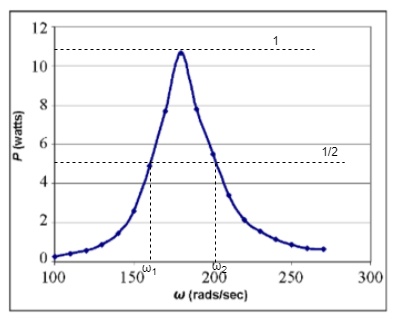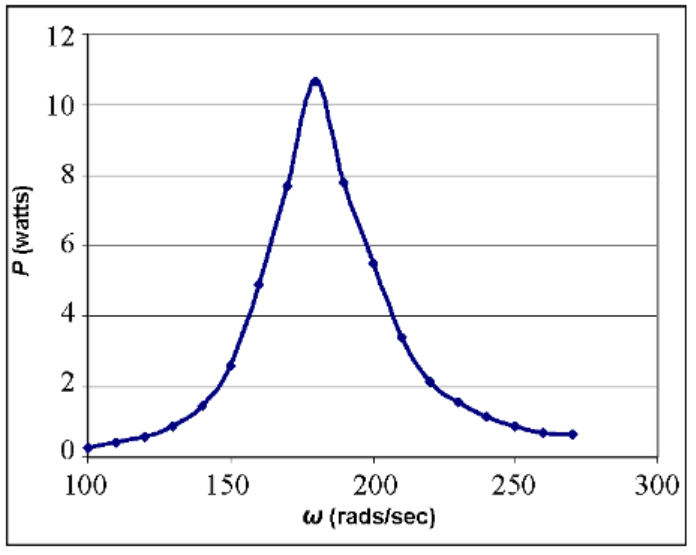# Problem: Use the graph below to determine quality factor Q. Express the answer with one decimal place.

###### FREE Expert Solution

Let's consider the diagram:Quality factor in the graph is given by:

Q = ω/Δω, where Δω = ω2 - ω1

86% (115 ratings)###### Problem Details

Use the graph below to determine quality factor Q. Express the answer with one decimal place.